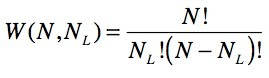## Sunday, March 14, 2010

### Illustrating Entropy

This screencast shows some Molecular Workbench models I made to illustrate entropy.

In the first model a monatomic gas consisting of 100 particles are initially restricted to the left container, but when the separator is removed the gas expands to fill both containers evenly, on average.

The temperature is held constant during the simulation, so the internal energy (which equal to the temperature times a constant) stays constant. The driving force behind the expansion is therefore purely entropic.

Using two simpler cases with two and three particles, I show that the probability of having NL particles (out of N total) on the left iswhere W is the weight or the number of equivalent combinations of particles in two containers. The screencast goes on to show that W is largest when the gas is evenly distributed between the two containers, so that is what happens when the separator is removed because that is the most probable thing to happen.

For N particles and two containers the number of combinations if there are NL particles in the left container is(as explained here and here).

As in the simpler cases, the most likely state for 100 particles is an equal distribution of particles among the two containers (NL = 50). The main difference between 3 and 100 particles is that in the former case you'll occasionally (2/8 or 25 % of the time) see all particles in one container, whereas for 100 particles you'll never (2 x 10-28 % of the time) see all particles in one container.

Entropy, S, is defined aswhere kB is Boltzmann's constant. This function is largest when W is largest, so one can say that the gas expands to maximize its entropy, but it's a bit like saying the gas expands because that is the most probable.

The change in entropy iswhich can be approximated using the more usual equation for the entropy change upon doubling the volume of an ideal gas while keeping the temperature constant(The latter equation becomes more accurate as the number of particles increase, because it relies on Stirling's approximation).

The model is too wide to fit in this blog but you can play around with it on this web page, or you can download the model if you have Molecular Workbench installed on your computer. Enjoy!

Related blog posts
Where does the ln come from in S = k ln(W)?
Entropy, volume, and temperature